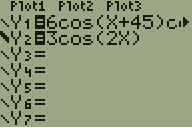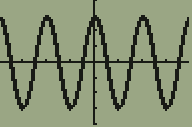# Proving trigonometric functions

• MHB
• kbr1804
In summary, the conversation discusses how to prove that $6\cos(x+45)\cos(x-45)$ is equal to $3\cos(2x)$, not $3\cos{x}$. The use of the sum/difference identity for cosine is mentioned and a typo is pointed out. Additional discussion on graphing this equation on Desmos is also included.

#### kbr1804

How can i prove that 6cos(x+45) cos(x-45) is equal to 3cosx?

use the sum/difference identity $\cos(a \pm b) = \cos{a}\cos{b} \mp \sin{a}\sin{b}$

it should be equal to $3\cos(2x)$, not $3\cos{x}$

Last edited by a moderator:
yeah i think i got it lol thanks alot:)and yeah it was supposed to equal to 3cos2x that was a typo

Beer soaked ramblings follow.
kbr1804 said:
How can i prove that 6cos(x+45) cos(x-45) is equal to 3cosx?
skeeter said:
use the sum/difference identity $\cos(a \pm b) = \cos{a}\cos{b} \mp \sin{a}\sin{b}$

it should be equal to $3\cos(2x)$, not $3\cos{x}$
kbr1804 said:
yeah i think i got it lol thanks alot:)and yeah it was supposed to equal to 3cos2x that was a typo
Three graphs; shouldn't 2 of them have the same graph?
https://www.desmos.com/calculator/x3dyyfbucy

show up the same on my calculator ...and on Desmos ...

[DESMOS]{"version":7,"graph":{"viewport":{"xmin":-180,"ymin":-10.762090536086637,"xmax":180,"ymax":10.762090536086637},"degreeMode":true,"squareAxes":false},"randomSeed":"78931067dd5aa2f77a194c669752ab59","expressions":{"list":[{"type":"expression","id":"1","color":"#c74440","latex":"6\\cos\\left(x+45\\right)\\cos\\left(x-45\\right)\\left\\{x>0\\right\\}"},{"type":"expression","id":"2","color":"#2d70b3","latex":"3\\cos\\left(2x\\right)\\left\\{x<0\\right\\}"},{"type":"expression","id":"3","color":"#388c46"}]}}[/DESMOS]

Last edited by a moderator:
jonah, in Desmos one should write $\pi/4$ instead of 45.

Evgeny.Makarov said:
jonah, in Desmos one should write $\pi/4$ instead of 45.

One can change to degree mode with the "wrench" button menu

Beer soaked ramblings follow.
Evgeny.Makarov said:
jonah, in Desmos one should write $\pi/4$ instead of 45.
skeeter said:
One can change to degree mode with the "wrench" button menu
Well aware of that.
Force of habit.
In the absence of the degree symbol (°), assumed that 45 was in radians.
Didn't occur to me to check it in degree mode.
Usually do it by multiplying the degree measure by $\frac{\pi}{180}$ if the expression just gives it once. Otherwise, I usually do it by skeeter's suggestion (which I'm also quite aware of).
skeeter said:
... and on Desmos ...

[DESMOS]{"version":7,"graph":{"viewport":{"xmin":-180,"ymin":-10.762090536086637,"xmax":180,"ymax":10.762090536086637},"degreeMode":true,"squareAxes":false},"randomSeed":"78931067dd5aa2f77a194c669752ab59","expressions":{"list":[{"type":"expression","id":"1","color":"#c74440","latex":"6\\cos\\left(x+45\\right)\\cos\\left(x-45\\right)\\left\\{x>0\\right\\}"},{"type":"expression","id":"2","color":"#2d70b3","latex":"3\\cos\\left(2x\\right)\\left\\{x<0\\right\\}"},{"type":"expression","id":"3","color":"#388c46"}]}}[/DESMOS]
I've often wondered what kind of platform this type of Desmos "quoting" is ever since I saw one of Klaas van Aarsen's post which used the same method. It isn't just a link to Desmos as I found out when I hit the reply tab on my phone. Is it that the TikZ thingamajig I've been seeing a lot lately on this site? I think I remember copying that stuff in another math site but was surprised that it didn't work there.

kbr1804 said:
How can i prove that 6cos(x+45) cos(x-45) is equal to 3cosx?
You CAN'T- it's not true! For example if x= 45 degrees this becomes 6 cos(90)cos(0)= 6(0)(1)= 0 but 3 cos(45)= 3sqrt(2)/2.

Country Boy said:
You CAN'T- it's not true! For example if x= 45 degrees this becomes 6 cos(90)cos(0)= 6(0)(1)= 0 but 3 cos(45)= 3sqrt(2)/2.

post #2 …

skeeter said:
use the sum/difference identity $\cos(a \pm b) = \cos{a}\cos{b} \mp \sin{a}\sin{b}$

it should be equal to $3\cos(2x)$, not $3\cos{x}$